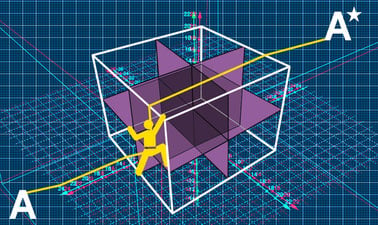# Imperial College London: A-Level Further Mathematics for Year 12 - Course 2: 3 x 3 Matrices, Mathematical Induction, Calculus Methods and Applications, Maclaurin Series, Complex Numbers and Polar Coordinates

Develop your thinking skills, fluency and confidence in A-level further maths and prepare for undergraduate STEM degrees.7 weeks
2–4 hours per week
Self-paced
Free

This course by Imperial College London is designed to help you develop the skills you need to succeed in your A-level further maths exams.

You will investigate key topic areas to gain a deeper understanding of the skills and techniques that you can apply throughout your A-level study. These skills include:

• Fluency – selecting and applying correct methods to answer with speed and efficiency
• Confidence – critically assessing mathematical methods and investigating ways to apply them
• Problem solving – analysing the ‘unfamiliar’ and identifying which skills and techniques you require to answer questions
• Constructing mathematical argument – using mathematical tools such as diagrams, graphs, logical deduction, mathematical symbols, mathematical language, construct mathematical argument and present precisely to others
• Deep reasoning – analysing and critiquing mathematical techniques, arguments, formulae and proofs to comprehend how they can be applied

Over eight modules, you will be introduced:

• The determinant and inverse of a 3 x 3 matrix
• Mathematical induction
• Differentiation and integration methods and some of their applications
• Maclaurin series
• DeMoivre’s Theorem for complex numbers and their applications
• Polar coordinates and sketching polar curves
• Hyperbolic functions

Your initial skillset will be extended to give a clear understanding of how background knowledge underpins the A-level further mathematics course. You’ll also, be encouraged to consider how what you know fits into the wider mathematical world.

### At a glance

• Institution: ImperialX
• Subject: Math
• Level: Intermediate
• Prerequisites:

An understanding of the content of the course A-Level Further Mathematics for Year 12: Course 1 is required

• Language: English
• Video Transcript: English
• Associated skills: Polar Coordinates, Sketching, Language Construct, Calculus, Integration

# What you'll learn

Skip What you'll learn

How to find the determinant of a complex number without using a calculator and interpret the result geometrically.
How to use properties of matrix determinants to simplify finding a determinant and to factorise determinants.
How to use a 3 x 3 matrix to apply a transformation in three dimensions
How to find the inverse of a 3 x 3 matrix without using a calculator.
How to prove series results using mathematical induction.
How to prove divisibility by mathematical induction.
How to prove matrix results by using mathematical induction.
How to use the chain, product and quotient rules for differentiation.
How to differentiate and integrate reciprocal and inverse trigonometric functions.
How to integrate by inspection.
How to use trigonometric identities to integrate.
How to use integration methods to find volumes of revolution.
How to use integration methods to find the mean of a function.
How to express functions as polynomial series.
How to find a Maclaurin series.
How to use standard Maclaurin series to define related series.
How to use De Moivre’s Theorem.
How to use polar coordinates to define a position in two dimensional space.
How to sketch the graphs of functions using polar coordinates.
How to define the hyperbolic sine and cosine of a value.
How to sketch graphs of hyperbolic functions.
How to differentiate and integrate hyperbolic functions.

# Syllabus

Skip Syllabus

Module 1: Matrices - The determinant and inverse of a 3 x 3 matrix

• Moving in to three dimensions
• Conventions for matrices in 3D
• The determinant of a 3 x 3 matrix and its geometrical interpretation
• Determinant properties
• Factorising a determinant
• Transformations using 3 x 3 matrices
• The inverse of a 3 x 3 matrix

Module 2: Mathematical induction

• The principle behind mathematical induction and the structure of proof by induction
• Mathematical induction and series
• Proving divisibility by induction
• Proving matrix results by induction

Module 3: Further differentiation and integration

• The chain rule
• The product rule and the quotient rule
• Differentiation of reciprocal and inverse trigonometric functions
• Integrating trigonometric functions
• Integrating functions that lead to inverse trigonometric integrals
• Integration by inspection
• Integration using trigonometric identities

Module 4: Applications of Integration

• Volumes of revolution
• The mean of a function

Module 5: An Introduction to Maclaurin series

• Expressing functions as polynomial series from first principles
• Maclaurin series

Module 6: Complex Numbers: De Moivre's Theorem and exponential form

• De Moivre's theorem and it's proof
• Using de Moivre’s Theorem to establish trigonometrical results
• De Moivre’s Theorem and complex exponents

Module 7: An introduction to polar coordinates

• Defining position using polar coordinates
• Sketching polar curves
• Cartesian to polar form and polar to Cartesian form

Module 8: Hyperbolic functions

• Defining hyperbolic functions
• Graphs of hyperbolic functions
• Calculations with hyperbolic functions
• Inverse hyperbolic functions

* Differentiating and integrating hyperbolic functions